This is the first chapter of Michael Nielsen's book **Neural Networ...
Nielsen strikes the perfect balance between the mechanics of neural...
Instead of exactly defining what a handwritten ***9*** is, and thin...
By "artificial neuron" he means the basic unit of computation upon ...
An **artificial neuron** is a mathematical function that mimics bio...
A perceptron is a computational model of a single neuron and it is ...
Alternatively we can use the [dot product](https://en.wikipedia.org...
This also called a [Weighted Average](https://en.wikipedia.org/wiki...
This is a simple example that perfectly illustrates the importance ...
Using the new notation where we introduce the bias, b: b = - \te...
In case you want to quickly freshen up your memory on logic gates: ...
We can implement a NAND gate using our perceptron function: ...
NAND gates and NOR gates are called Universal Gates because you can...
Perceptrons use learning algorithms to automatically tune the weigh...
Functions for which a small change in input causes a bounded change...
Perceptrons are not smooth, continuous functions and therefore not ...
[Pierre François Verhulst](https://en.wikipedia.org/wiki/Pierre_Fra...
In Python: py import math def sigmoid(x): return 1 / (...
The perceptron is an extreme case of a sigmoid neutron when the abs...
Notice that $\sigma(z)$ approaches $1$ as $z$ grows towards $\infty... Even though you can see a vertical, continuous line on the graph, a... Unlike the perceptron, the sigmoid is continuous and differentiable... The Sigmoid is an activation function of the neural network. Some o... It is called an "activation function" because it determines for whi... In a binary classification (true, or false`), we can also interp... Let's start by showing that the formula for any given perceptron do... Lets first remember what happens to$\sigma(z)$when its input grow... In this context, the way in which the neurons are connected to each... The figure below shows an example of an image with 9 pixels, each p... **The Input Layer:** A neural network has exactly one input layer ... The distinction between feedforward and feedback is also found in [... In a feedforward neural network every unit in of the layer is conne... The heuristic techniques of neural net designs include such ideas a... Let's start thinking about what we want to find: the weights$w_{ij...
Here is a video of Stanford professor Andrew Ng explaining gradient...
**A training set** is a data set used to discover predictive relati...
Even though we think about the image as a 2D $28 \times 28$ vector,...
Here is a video of Stanford professor Andrew Ng explaining what a c...
[Cost functions](https://en.wikipedia.org/wiki/Cost_function) are u...
The cost functions help determine and refine the weights W, and bia...
Here is a video with one of my favourite explanations of gradient d...
By "calculus doesn't work" the author means that finding the closed...
The change of $C$, $\Delta C$, is the sum of the change in each dir...
This is a linear approximation that is only valid around one point....
The value of $\eta$ is important: - if $\eta$ is too small gradien...
Our goal is to pick in which direction we are going to step, \$\Delt...
If one dimension, we can think about it as a cart moving through a ...
The intuition is the following: 1. We know the direction in which...
Stochaistic: randomly determined.
Epoch: period in time. In this context, a step.
One advantage comes in a "live context". As the model is operating ...
The batches are randomized for the same reason arrays are shuffled ...
This [Tensor Flow tutorial](https://www.tensorflow.org/versions/r0....
I think about the hyper-parameters as the parameters that are used ...
Though useful, the comparison between function abstraction in progr...The human visual system is one of the wonders of the world.
Consider the following sequence of handwritten digits:
Most people effortlessly recognize those digits as 504192. That
ease is deceptive. In each hemisphere of our brain, humans
have a primary visual cortex, also known as V1, containing 140
million neurons, with tens of billions of connections between
them. And yet human vision involves not just V1, but an entire
series of visual cortices - V2, V3, V4, and V5 - doing
progressively more complex image processing. We carry in our
heads a supercomputer, tuned by evolution over hundreds of
millions of years, and superbly adapted to understand the
visual world. Recognizing handwritten digits isn't easy. Rather,
we humans are stupendously, astoundingly good at making
sense of what our eyes show us. But nearly all that work is done
unconsciously. And so we don't usually appreciate how tough a
problem our visual systems solve.
The difficulty of visual pattern recognition becomes apparent if
you attempt to write a computer program to recognize digits
like those above. What seems easy when we do it ourselves
suddenly becomes extremely difficult. Simple intuitions about
how we recognize shapes - "a 9 has a loop at the top, and a
vertical stroke in the bottom right" - turn out to be not so
simple to express algorithmically. When you try to make such
rules precise, you quickly get lost in a morass of exceptions and
caveats and special cases. It seems hopeless.
Neural networks approach the problem in a different way. The
idea is to take a large number of handwritten digits, known as
training examples,
CHAPTER 1
Using neural nets to recognize handwritten digitsand then develop a system which can learn from those training
examples. In other words, the neural network uses the
examples to automatically infer rules for recognizing
handwritten digits. Furthermore, by increasing the number of
handwriting, and so improve its accuracy. So while I've shown
just 100 training digits above, perhaps we could build a better
handwriting recognizer by using thousands or even millions or
billions of training examples.
In this chapter we'll write a computer program implementing a
neural network that learns to recognize handwritten digits. The
program is just 74 lines long, and uses no special neural
network libraries. But this short program can recognize digits
with an accuracy over 96 percent, without human intervention.
Furthermore, in later chapters we'll develop ideas which can
improve accuracy to over 99 percent. In fact, the best
commercial neural networks are now so good that they are
used by banks to process cheques, and by post offices to
We're focusing on handwriting recognition because it's an
excellent prototype problem for learning about neural
networks in general. As a prototype it hits a sweet spot: it's
challenging - it's no small feat to recognize handwritten digits -
but it's not so difficult as to require an extremely complicated
solution, or tremendous computational power. Furthermore,
it's a great way to develop more advanced techniques, such as
deep learning. And so throughout the book we'll return
repeatedly to the problem of handwriting recognition. Later in
the book, we'll discuss how these ideas may be applied to otherproblems in computer vision, and also in speech, natural
language processing, and other domains.
Of course, if the point of the chapter was only to write a
computer program to recognize handwritten digits, then the
chapter would be much shorter! But along the way we'll
develop many key ideas about neural networks, including two
important types of artificial neuron (the perceptron and the
sigmoid neuron), and the standard learning algorithm for
neural networks, known as stochastic gradient descent.
Throughout, I focus on explaining why things are done the way
they are, and on building your neural networks intuition. That
requires a lengthier discussion than if I just presented the basic
mechanics of what's going on, but it's worth it for the deeper
understanding you'll attain. Amongst the payoffs, by the end of
the chapter we'll be in position to understand what deep
learning is, and why it matters.
Perceptrons
What is a neural network? To get started, I'll explain a type of
artificial neuron called a perceptron. Perceptrons were
developed in the 1950s and 1960s by the scientist Frank
Rosenblatt, inspired by earlier work by Warren McCulloch and
Walter Pitts. Today, it's more common to use other models of
artificial neurons - in this book, and in much modern work on
neural networks, the main neuron model used is one called the
sigmoid neuron. We'll get to sigmoid neurons shortly. But to
understand why sigmoid neurons are defined the way they are,
it's worth taking the time to first understand perceptrons.
So how do perceptrons work? A perceptron takes several
binary inputs, , and produces a single binary output:
In the example shown the perceptron has three inputs,
. In general it could have more or fewer inputs.
Rosenblatt proposed a simple rule to compute the output. He
introduced weights, , real numbers expressing the
importance of the respective inputs to the output. The neuron's
, ,
x
1
x
2
, ,
x
1
x
2
x
3
, ,
w
1
w
2output, or , is determined by whether the weighted sum
is less than or greater than some threshold value. Just
like the weights, the threshold is a real number which is a
parameter of the neuron. To put it in more precise algebraic
terms:
That's all there is to how a perceptron works!
That's the basic mathematical model. A way you can think
about the perceptron is that it's a device that makes decisions
by weighing up evidence. Let me give an example. It's not a
very realistic example, but it's easy to understand, and we'll
soon get to more realistic examples. Suppose the weekend is
coming up, and you've heard that there's going to be a cheese
festival in your city. You like cheese, and are trying to decide
whether or not to go to the festival. You might make your
decision by weighing up three factors:
1. Is the weather good?
2. Does your boyfriend or girlfriend want to accompany you?
3. Is the festival near public transit? (You don't own a car).
We can represent these three factors by corresponding binary
variables , and . For instance, we'd have if the
weather is good, and if the weather is bad. Similarly,
if your boyfriend or girlfriend wants to go, and if
not. And similarly again for and public transit.
Now, suppose you absolutely adore cheese, so much so that
you're happy to go to the festival even if your boyfriend or
girlfriend is uninterested and the festival is hard to get to. But
perhaps you really loathe bad weather, and there's no way
you'd go to the festival if the weather is bad. You can use
perceptrons to model this kind of decision-making. One way to
do this is to choose a weight for the weather, and
and for the other conditions. The larger value of
indicates that the weather matters a lot to you, much more
than whether your boyfriend or girlfriend joins you, or the
nearness of public transit. Finally, suppose you choose a
threshold of for the perceptron. With these choices, the
perceptron implements the desired decision-making model,
0 1
j
w
j
x
j
output
=
{
0
1
if threshold
j
w
j
x
j
if > threshold
j
w
j
x
j
(1)
,
x
1
x
2
x
3
= 1
x
1
= 0
x
1
= 1
x
2
= 0
x
2
x
3
= 6
w
1
= 2
w
2
= 2
w
3
w
1
5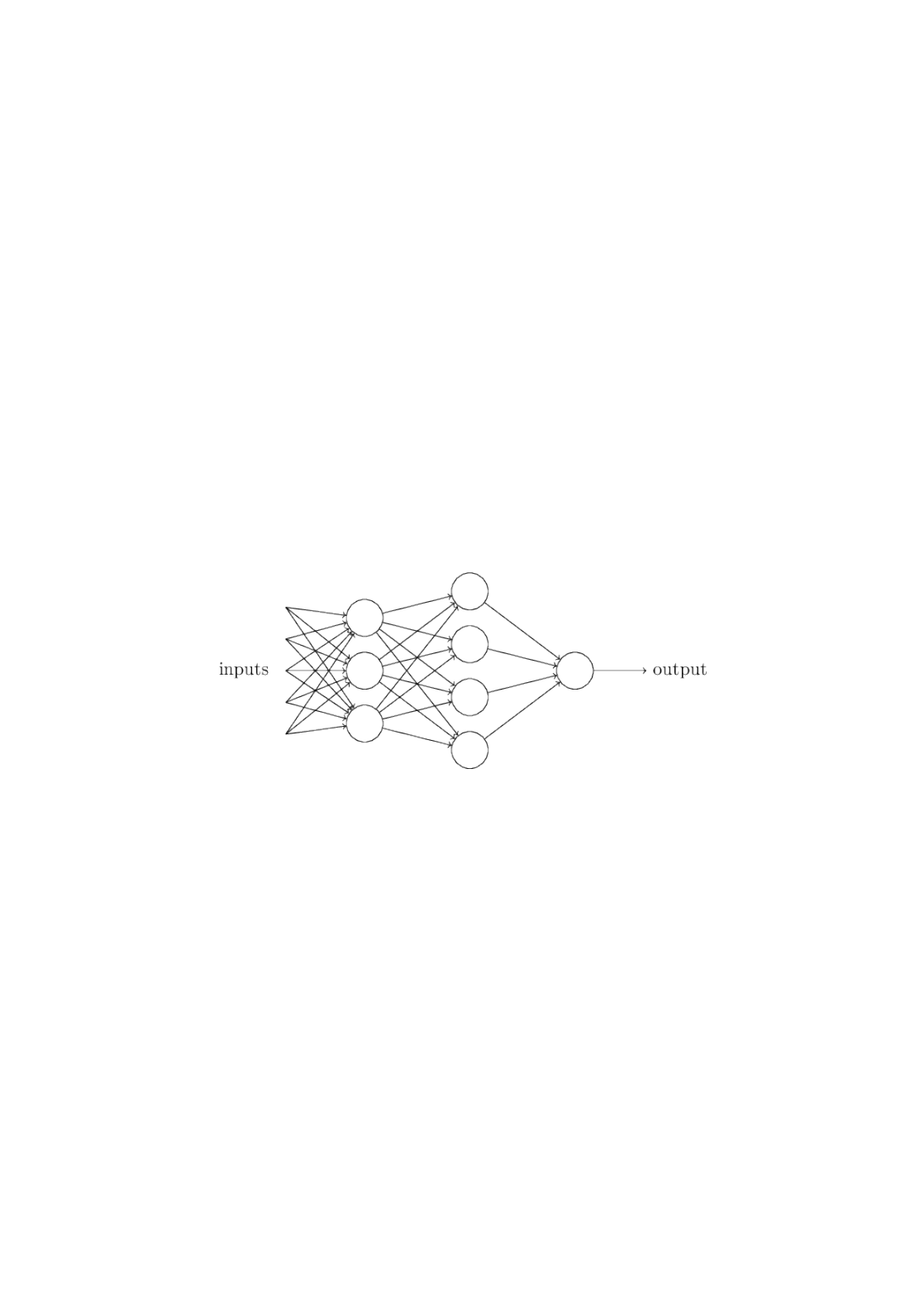outputting whenever the weather is good, and whenever the
weather is bad. It makes no difference to the output whether
your boyfriend or girlfriend wants to go, or whether public
transit is nearby.
By varying the weights and the threshold, we can get different
models of decision-making. For example, suppose we instead
chose a threshold of . Then the perceptron would decide that
you should go to the festival whenever the weather was good or
when both the festival was near public transit and your
boyfriend or girlfriend was willing to join you. In other words,
it'd be a different model of decision-making. Dropping the
threshold means you're more willing to go to the festival.
Obviously, the perceptron isn't a complete model of human
decision-making! But what the example illustrates is how a
perceptron can weigh up different kinds of evidence in order to
make decisions. And it should seem plausible that a complex
network of perceptrons could make quite subtle decisions:
In this network, the first column of perceptrons - what we'll call
the first layer of perceptrons - is making three very simple
decisions, by weighing the input evidence. What about the
perceptrons in the second layer? Each of those perceptrons is
making a decision by weighing up the results from the first
layer of decision-making. In this way a perceptron in the
second layer can make a decision at a more complex and more
abstract level than perceptrons in the first layer. And even
more complex decisions can be made by the perceptron in the
third layer. In this way, a many-layer network of perceptrons
can engage in sophisticated decision making.
Incidentally, when I defined perceptrons I said that a
perceptron has just a single output. In the network above the
perceptrons look like they have multiple outputs. In fact,
they're still single output. The multiple output arrows are
merely a useful way of indicating that the output from a
1 0
3perceptron is being used as the input to several other
perceptrons. It's less unwieldy than drawing a single output
line which then splits.
Let's simplify the way we describe perceptrons. The condition
is cumbersome, and we can make two
notational changes to simplify it. The first change is to write
as a dot product, , where and are
vectors whose components are the weights and inputs,
respectively. The second change is to move the threshold to the
other side of the inequality, and to replace it by what's known
as the perceptron's bias, . Using the bias instead
of the threshold, the perceptron rule can be rewritten:
You can think of the bias as a measure of how easy it is to get
the perceptron to output a . Or to put it in more biological
terms, the bias is a measure of how easy it is to get the
perceptron to fire. For a perceptron with a really big bias, it's
extremely easy for the perceptron to output a . But if the bias
is very negative, then it's difficult for the perceptron to output a
. Obviously, introducing the bias is only a small change in how
we describe perceptrons, but we'll see later that it leads to
further notational simplifications. Because of this, in the
remainder of the book we won't use the threshold, we'll always
use the bias.
I've described perceptrons as a method for weighing evidence
to make decisions. Another way perceptrons can be used is to
compute the elementary logical functions we usually think of as
underlying computation, functions such as AND, OR, and NAND.
For example, suppose we have a perceptron with two inputs,
each with weight , and an overall bias of . Here's our
perceptron:
Then we see that input produces output , since
is positive. Here, I've introduced the
symbol to make the multiplications explicit. Similar
calculations show that the inputs and produce output .
> threshold
j
w
j
x
j
j
w
j
x
j
w x
j
w
j
x
j
w x
b threshold
output =
{
0
1
if w x + b 0
if w x + b > 0
(2)
1
1
1
2 3
00 1
(2) 0 + (2) 0 + 3 = 3
01 10 1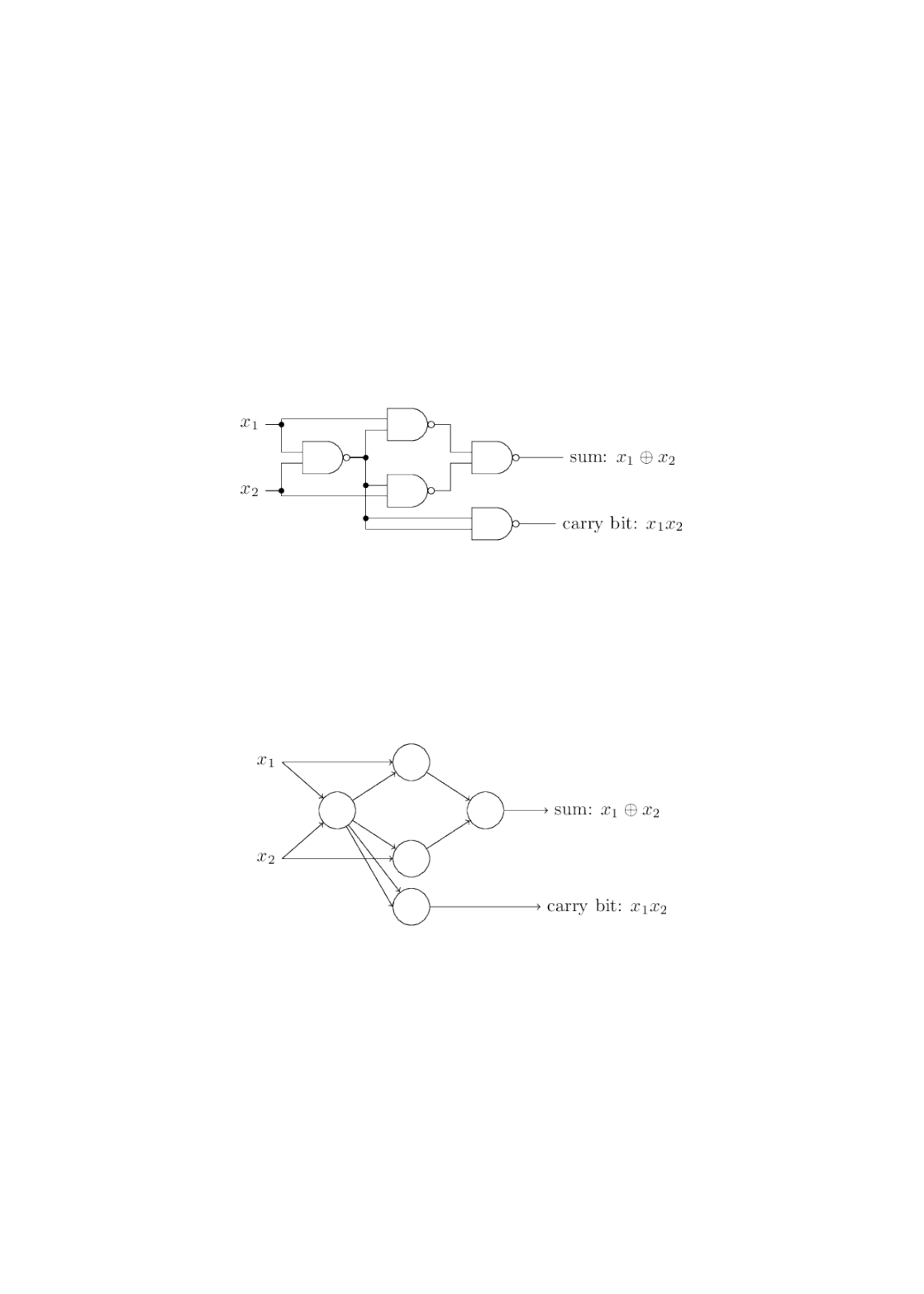But the input produces output , since
is negative. And so our perceptron
implements a NAND gate!
The NAND example shows that we can use perceptrons to
compute simple logical functions. In fact, we can use networks
of perceptrons to compute any logical function at all. The
reason is that the NAND gate is universal for computation, that
is, we can build any computation up out of NAND gates. For
example, we can use NAND gates to build a circuit which adds
two bits, and . This requires computing the bitwise sum,
, as well as a carry bit which is set to when both and
are , i.e., the carry bit is just the bitwise product :
To get an equivalent network of perceptrons we replace all the
NAND gates by perceptrons with two inputs, each with weight
, and an overall bias of . Here's the resulting network. Note
that I've moved the perceptron corresponding to the bottom
right NAND gate a little, just to make it easier to draw the
arrows on the diagram:
One notable aspect of this network of perceptrons is that the
output from the leftmost perceptron is used twice as input to
the bottommost perceptron. When I defined the perceptron
model I didn't say whether this kind of double-output-to-the-
same-place was allowed. Actually, it doesn't much matter. If we
don't want to allow this kind of thing, then it's possible to
simply merge the two lines, into a single connection with a
weight of -4 instead of two connections with -2 weights. (If you
don't find this obvious, you should stop and prove to yourself
that this is equivalent.) With that change, the network looks as
follows, with all unmarked weights equal to -2, all biases equal
11 0
(2) 1 + (2) 1 + 3 = 1
x
1
x
2
x
1
x
2
1
x
1
x
2
1
x
1
x
2
2 3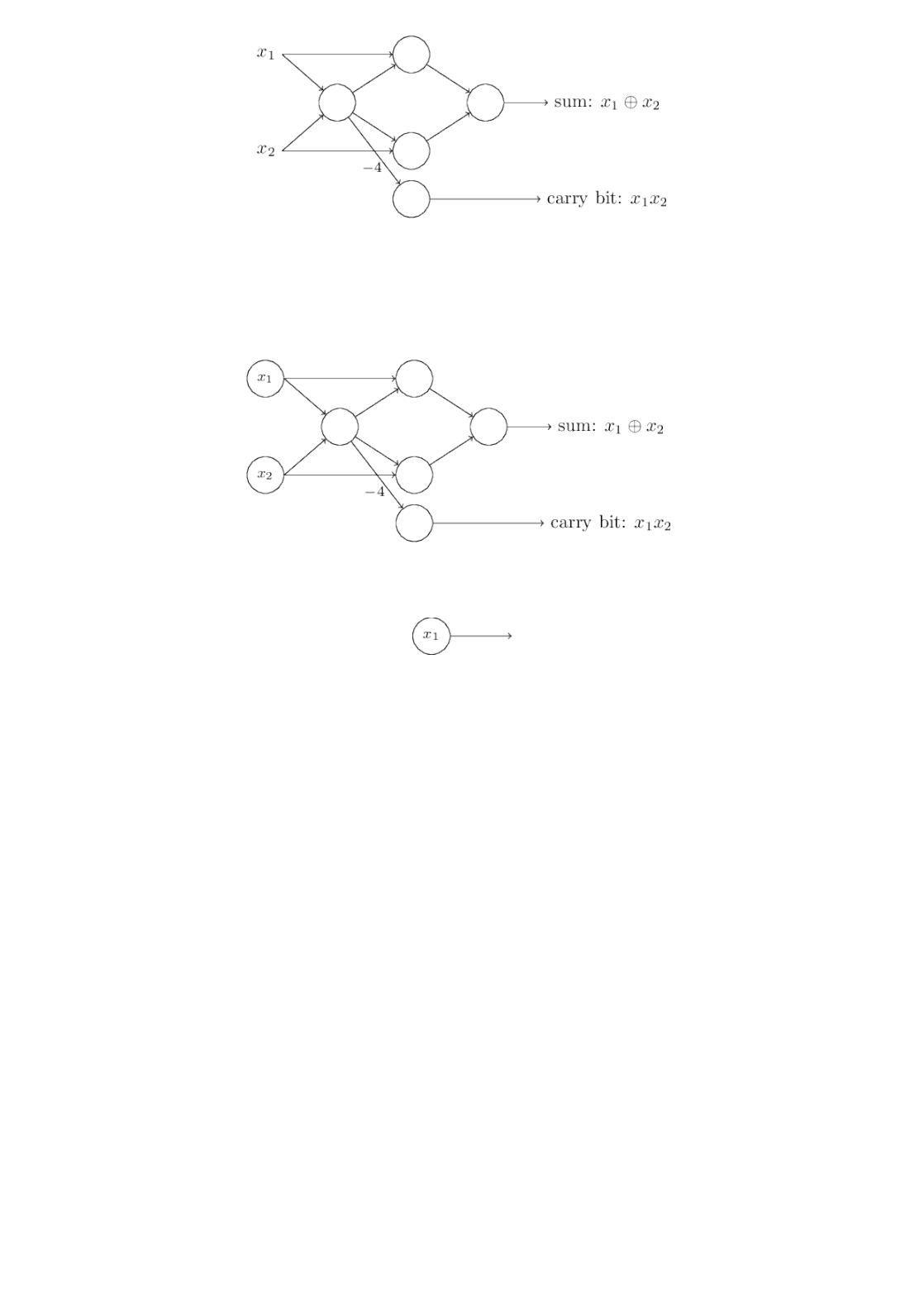to 3, and a single weight of -4, as marked:
Up to now I've been drawing inputs like and as variables
floating to the left of the network of perceptrons. In fact, it's
conventional to draw an extra layer of perceptrons - the input
layer - to encode the inputs:
This notation for input perceptrons, in which we have an
output, but no inputs,
is a shorthand. It doesn't actually mean a perceptron with no
inputs. To see this, suppose we did have a perceptron with no
inputs. Then the weighted sum would always be zero,
and so the perceptron would output if , and if .
That is, the perceptron would simply output a fixed value, not
the desired value ( , in the example above). It's better to think
of the input perceptrons as not really being perceptrons at all,
but rather special units which are simply defined to output the
desired values, .
The adder example demonstrates how a network of
perceptrons can be used to simulate a circuit containing many
NAND gates. And because NAND gates are universal for
computation, it follows that perceptrons are also universal for
computation.
The computational universality of perceptrons is
simultaneously reassuring and disappointing. It's reassuring
because it tells us that networks of perceptrons can be as
powerful as any other computing device. But it's also
disappointing, because it makes it seem as though perceptrons
x
1
x
2
j
w
j
x
j
1 b > 0 0
b 0
x
1
, ,
x
1
x
2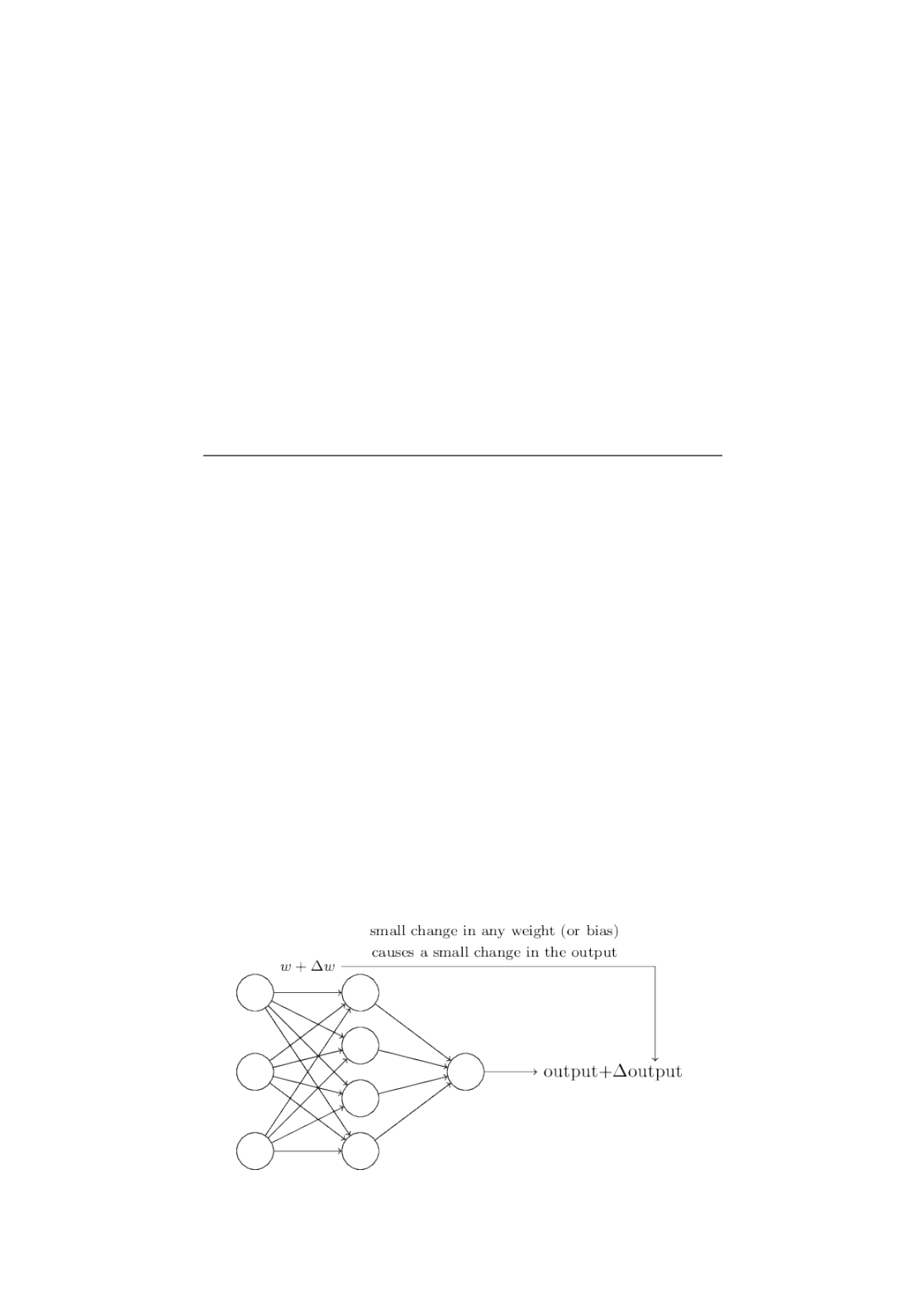are merely a new type of NAND gate. That's hardly big news!
However, the situation is better than this view suggests. It
turns out that we can devise learning algorithms which can
automatically tune the weights and biases of a network of
artificial neurons. This tuning happens in response to external
stimuli, without direct intervention by a programmer. These
learning algorithms enable us to use artificial neurons in a way
of explicitly laying out a circuit of NAND and other gates, our
neural networks can simply learn to solve problems, sometimes
problems where it would be extremely difficult to directly
design a conventional circuit.
Sigmoid neurons
Learning algorithms sound terrific. But how can we devise such
algorithms for a neural network? Suppose we have a network of
perceptrons that we'd like to use to learn to solve some
problem. For example, the inputs to the network might be the
raw pixel data from a scanned, handwritten image of a digit.
And we'd like the network to learn weights and biases so that
the output from the network correctly classifies the digit. To
see how learning might work, suppose we make a small change
in some weight (or bias) in the network. What we'd like is for
this small change in weight to cause only a small corresponding
change in the output from the network. As we'll see in a
moment, this property will make learning possible.
Schematically, here's what we want (obviously this network is
too simple to do handwriting recognition!):
If it were true that a small change in a weight (or bias) causes
only a small change in output, then we could use this fact to
modify the weights and biases to get our network to behavemore in the manner we want. For example, suppose the
network was mistakenly classifying an image as an "8" when it
should be a "9". We could figure out how to make a small
change in the weights and biases so the network gets a little
closer to classifying the image as a "9". And then we'd repeat
this, changing the weights and biases over and over to produce
better and better output. The network would be learning.
The problem is that this isn't what happens when our network
contains perceptrons. In fact, a small change in the weights or
bias of any single perceptron in the network can sometimes
cause the output of that perceptron to completely flip, say from
to . That flip may then cause the behaviour of the rest of the
network to completely change in some very complicated way.
So while your "9" might now be classified correctly, the
behaviour of the network on all the other images is likely to
have completely changed in some hard-to-control way. That
makes it difficult to see how to gradually modify the weights
and biases so that the network gets closer to the desired
behaviour. Perhaps there's some clever way of getting around
this problem. But it's not immediately obvious how we can get
a network of perceptrons to learn.
We can overcome this problem by introducing a new type of
artificial neuron called a sigmoid neuron. Sigmoid neurons are
similar to perceptrons, but modified so that small changes in
their weights and bias cause only a small change in their
output. That's the crucial fact which will allow a network of
sigmoid neurons to learn.
Okay, let me describe the sigmoid neuron. We'll depict sigmoid
neurons in the same way we depicted perceptrons:
Just like a perceptron, the sigmoid neuron has inputs,
. But instead of being just or , these inputs can also
take on any values between and . So, for instance, is
a valid input for a sigmoid neuron. Also just like a perceptron,
the sigmoid neuron has weights for each input, , and
an overall bias, . But the output is not or . Instead, it's
0 1
, ,
x
1
x
2
0 1
0 1 0.638
, ,
w
1
w
2
b 0 1, where is called the sigmoid function*
*Incidentally, is sometimes called the logistic function, and this new class of
neurons called logistic neurons. It's useful to remember this terminology,
since these terms are used by many people working with neural nets. However,
we'll stick with the sigmoid terminology.
, and is defined by:
To put it all a little more explicitly, the output of a sigmoid
neuron with inputs , weights , and bias is
At first sight, sigmoid neurons appear very different to
perceptrons. The algebraic form of the sigmoid function may
seem opaque and forbidding if you're not already familiar with
it. In fact, there are many similarities between perceptrons and
sigmoid neurons, and the algebraic form of the sigmoid
function turns out to be more of a technical detail than a true
barrier to understanding.
To understand the similarity to the perceptron model, suppose
is a large positive number. Then and so
. In other words, when is large and
positive, the output from the sigmoid neuron is approximately
, just as it would have been for a perceptron. Suppose on the
other hand that is very negative. Then ,
and . So when is very negative, the
behaviour of a sigmoid neuron also closely approximates a
perceptron. It's only when is of modest size that
there's much deviation from the perceptron model.
What about the algebraic form of ? How can we understand
that? In fact, the exact form of isn't so important - what really
matters is the shape of the function when plotted. Here's the
shape:
σ(w x + b)
σ
σ
σ(z) .
1
1 +
e
z
(3)
, ,
x
1
x
2
, ,
w
1
w
2
b
.
1
1 + exp( b)
j
w
j
x
j
(4)
z w x + b
0
e
z
σ(z) 1
z = w x + b
1
z = w x + b
e
z
σ(z) 0
z = w x + b
w x + b
σ
σ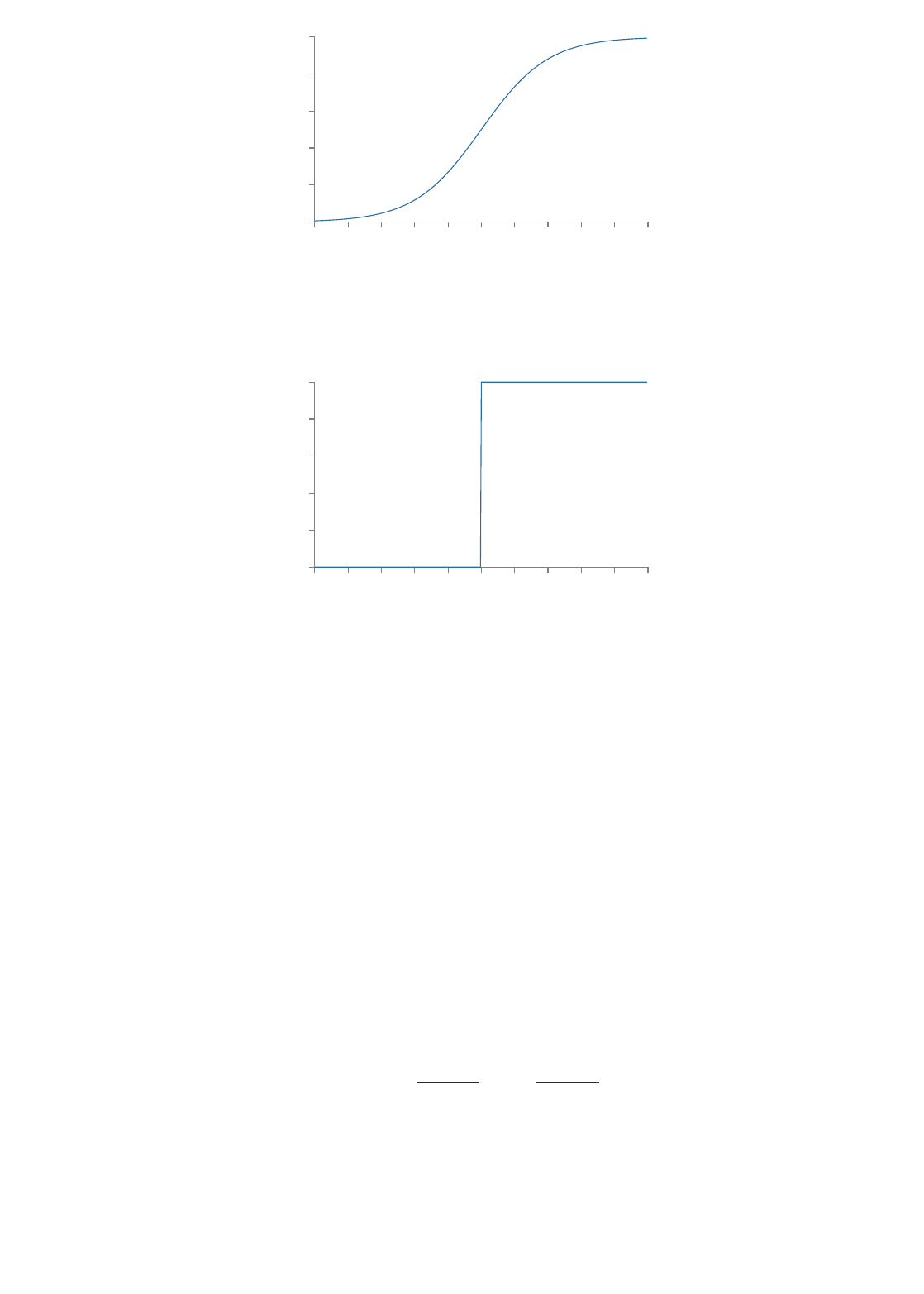-4 -3 -2 -1 0 1 2 3 4
0.0
0.2
0.4
0.6
0.8
1.0
z
sigmoid function
This shape is a smoothed out version of a step function:
-4 -3 -2 -1 0 1 2 3 4
0.0
0.2
0.4
0.6
0.8
1.0
z
step function
If had in fact been a step function, then the sigmoid neuron
would be a perceptron, since the output would be or
depending on whether was positive or negative*
*Actually, when the perceptron outputs , while the step function
outputs . So, strictly speaking, we'd need to modify the step function at that
one point. But you get the idea.
. By using the actual function we get, as already implied
above, a smoothed out perceptron. Indeed, it's the smoothness
of the function that is the crucial fact, not its detailed form.
The smoothness of means that small changes in the
weights and in the bias will produce a small change
in the output from the neuron. In fact, calculus tells us that
is well approximated by
where the sum is over all the weights, , and and
denote partial derivatives of the with respect
to and , respectively. Don't panic if you're not comfortable
with partial derivatives! While the expression above looks
complicated, with all the partial derivatives, it's actually saying
σ
1 0
w x + b
w x + b = 0
0
1
σ
σ
σ
Δ
w
j
Δb
Δoutput
Δoutput
Δoutput Δ + Δb,
j
output
w
j
w
j
output
b
(5)
w
j
output/
w
j
output/b
output
w
j
bsomething very simple (and which is very good news):
is a linear function of the changes and in the weights
and bias. This linearity makes it easy to choose small changes
in the weights and biases to achieve any desired small change
in the output. So while sigmoid neurons have much of the same
qualitative behaviour as perceptrons, they make it much easier
to figure out how changing the weights and biases will change
the output.
If it's the shape of which really matters, and not its exact
form, then why use the particular form used for in Equation
(3)? In fact, later in the book we will occasionally consider
neurons where the output is for some other
activation function . The main thing that changes when we
use a different activation function is that the particular values
for the partial derivatives in Equation (5) change. It turns out
that when we compute those partial derivatives later, using
will simplify the algebra, simply because exponentials have
lovely properties when differentiated. In any case, is
commonly-used in work on neural nets, and is the activation
function we'll use most often in this book.
How should we interpret the output from a sigmoid neuron?
Obviously, one big difference between perceptrons and sigmoid
neurons is that sigmoid neurons don't just output or . They
can have as output any real number between and , so values
such as and are legitimate outputs. This can
be useful, for example, if we want to use the output value to
represent the average intensity of the pixels in an image input
to a neural network. But sometimes it can be a nuisance.
Suppose we want the output from the network to indicate
either "the input image is a 9" or "the input image is not a 9".
Obviously, it'd be easiest to do this if the output was a or a ,
as in a perceptron. But in practice we can set up a convention
to deal with this, for example, by deciding to interpret any
output of at least as indicating a "9", and any output less
than as indicating "not a 9". I'll always explicitly state when
we're using such a convention, so it shouldn't cause any
confusion.
Exercises
Sigmoid neurons simulating perceptrons, part I
Δoutput
Δ
w
j
Δb
σ
σ
f (w x + b)
f ()
σ
σ
0 1
0 1
0.173 0.689
0 1
0.5
0.5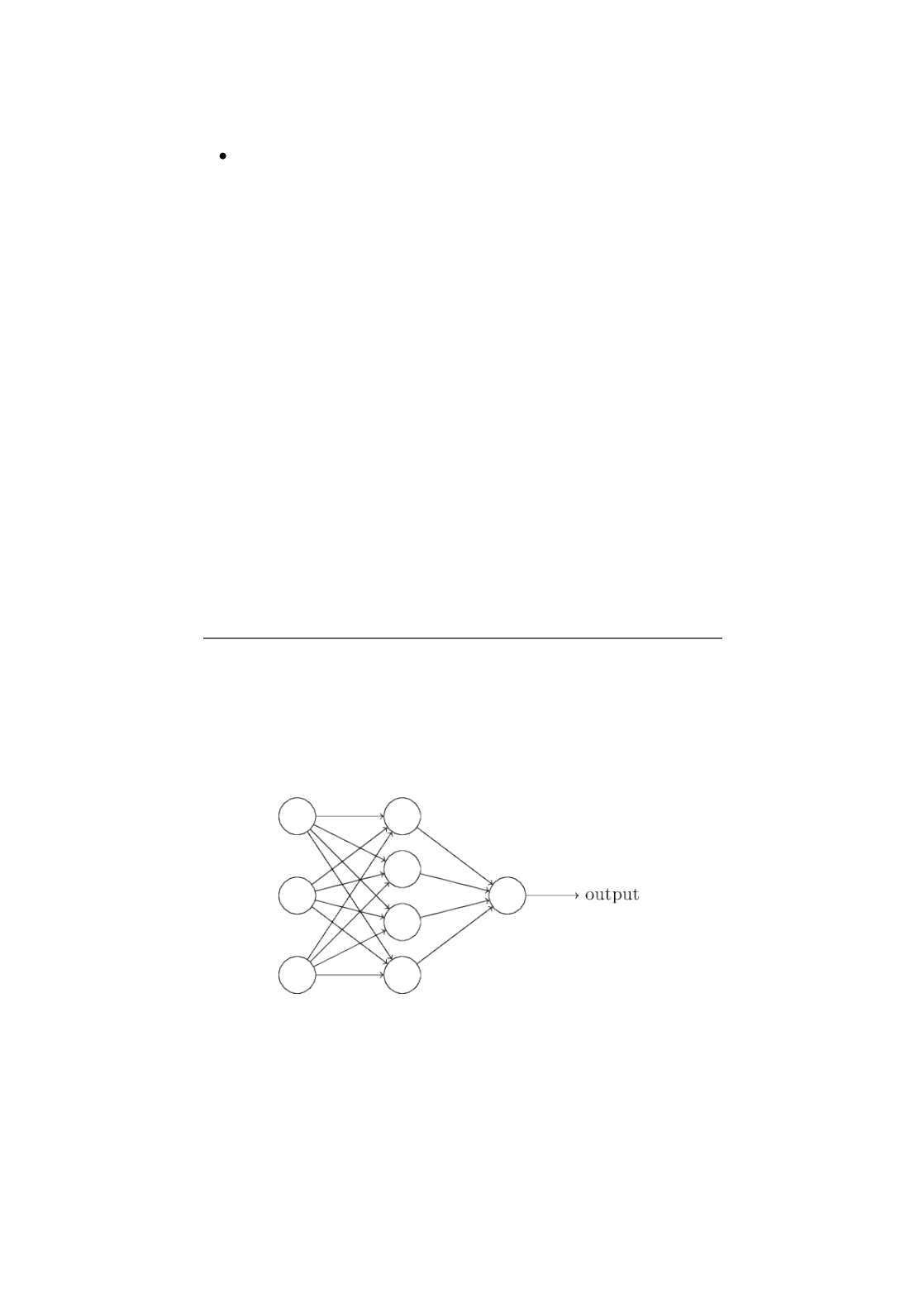Suppose we take all the weights and biases in a network of
perceptrons, and multiply them by a positive constant,
. Show that the behaviour of the network doesn't
change.
Sigmoid neurons simulating perceptrons, part II
Suppose we have the same setup as the last problem - a
network of perceptrons. Suppose also that the overall
input to the network of perceptrons has been chosen. We
won't need the actual input value, we just need the input to
have been fixed. Suppose the weights and biases are such
that for the input to any particular
perceptron in the network. Now replace all the
perceptrons in the network by sigmoid neurons, and
multiply the weights and biases by a positive constant
. Show that in the limit as the behaviour of
this network of sigmoid neurons is exactly the same as the
network of perceptrons. How can this fail when
for one of the perceptrons?
The architecture of neural networks
In the next section I'll introduce a neural network that can do a
pretty good job classifying handwritten digits. In preparation
for that, it helps to explain some terminology that lets us name
different parts of a network. Suppose we have the network:
As mentioned earlier, the leftmost layer in this network is
called the input layer, and the neurons within the layer are
called input neurons. The rightmost or output layer contains
the output neurons, or, as in this case, a single output neuron.
The middle layer is called a hidden layer, since the neurons in
this layer are neither inputs nor outputs. The term "hidden"
perhaps sounds a little mysterious - the first time I heard the
term I thought it must have some deep philosophical or
mathematical significance - but it really means nothing more
c > 0
w x + b 0
x
c > 0
c
w x +